# Modeling the Budding Yeast Cell Cycle

## Equations

A description of how the equations are formulated is presented in Chen et al., 2004.

Equation for growth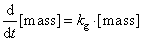Equations governing cyclin-dependent kinases:Equations governing the inhibitors of Clb-dependent kinases:Equations governing Clb degradation machinery:Equations for the MEN pathway:Equations governing the phosphatases: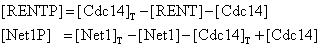Equations for Pds1, Esp1 and their interaction:Equations for DNA synthesis, budding and spindle formation:Goldbeter Function: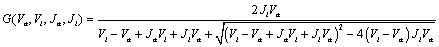Equations governing the transcription factors: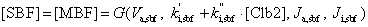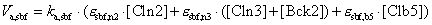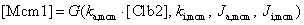Reset Rules:

When [Clb2] drops belowas cell exits mitosis, we reset [BUD] and [SPN] to zero, and divide the mass between daughter and mother cells as follows: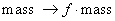for daughter, and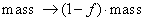for mother, with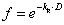, where D is the daughter cycle time. Lord & Wheals, 1980 found empirically that D and growth rateare related by the equation,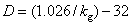. For a culture growing at a mass-doubling-time of 90 min,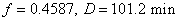. When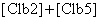drops below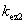, [ORI] is reset to 0.

Flags:  Bud emergence when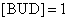, start DNA synthesis when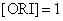, chromosome alignment on spindle completed when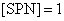.